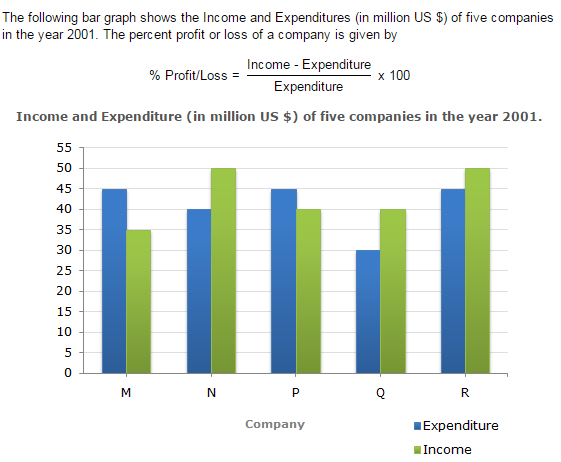Direction1.

The companies M and N together had a percentage of profit/loss of?

 A. 12% loss B. 10% loss C. 10% profit D. There was no loss or profit
Explanation: Total income of Companies M and N together = (35 + 50) million US \$ = 85 million US \$ Total expenditure of Companies M and N together = (45 + 40) million US \$ = 85 million US \$. Therefore Percent Profit/Loss of companies M and N together % Profit/Loss = ( 85 - 85 x 100 ) = 0%. 85 Thus, there was neither loss nor profit for companies M and N together.

2.

In 2001, what was the approximate percentage of profit/loss of all the five Companies taken together?

 A. 5% profit B. 6.5% profit C. 4% loss D. 7% loss
Explanation: Total income of all five companies = (35 + 50 + 40 + 40 + 50) million US \$ = 215 million US \$. Total expenditure of all five companies = (45 + 40 + 45 + 30 + 45) million US \$ = 205 million US \$. Therefore % Profit = [ (215 - 205) x 100 ] % = 4.88% ~= 5%. 205

3.

Which company earned the maximum percentage profit in the year 2001?

 A. M B. N C. P D. Q
Explanation: The percentage profit/loss in the year 2001 for various comapanies are: For M = [ (35 - 45) x 100 ] % = -22.22% i.e., Loss = 22.22%. 45 For N = [ (50 - 40) x 100 ] % = 25% i.e., Profit = 25%. 40 For P = [ (40 - 45) x 100 ] % = -11.11% i.e., Loss = 11.11%. 45 For Q = [ (40 - 30) x 100 ] % = 33.33% i.e., Profit = 33.33%. 30 For R = [ (50 - 45) x 100 ] % = 11.11% i.e., Profit = 11.11%. 45 Clearly, the Company Q earned the maximum profit in 2001.

4.

For Company R, if the expenditure had increased by 20% in year 2001 from year 2000 and the company had earned profit of 10% in 2000, what was the Company's income in 2000 (in million US \$)?

 A. 35.75 B. 37.25 C. 38.5 D. 41.25